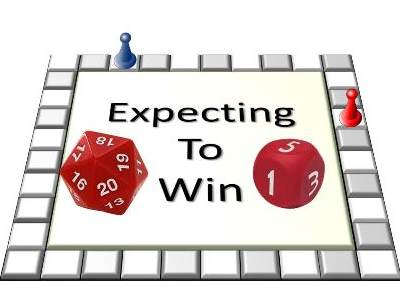# Activities

•• ##### AuthorAust Senior

50 Minutes

• ##### Device
•TI-Nspire™ CX
•TI-Nspire™ CX CAS
• ##### Software

TI-Nspire™
TI-Nspire™ CAS

4.5

## Expecting a Win#### Activity Overview

Many board games require players to land precisely on the last square of the board in order to complete the game. This investigation involves the simplest of board games. Players take it in turns to roll a die, each time advancing toward the final square. Players must finish precisely on the last square in order to finish the game. So far the game sounds simple and fair. There is however one catch! Players can nominate the number of ‘sides’ on their die. As the die is virtual (simulated by the calculator), players can nominate any quantity between 1 and 20. There are 20 squares on the board, so exactly 20 squares must be advanced and the first player to do so is the winner!

#### Objectives

• Use simulations to estimate probabilities
• Understanding of Central Limit Theorem (Informal)
• Calculate mean and standard deviation of a sample (Using technology)
• Understand difference between mean, median and mean
• Solve problems using simulations

#### Vocabulary

• Mean, Mode, Median, Expected Value
• Standard Deviation
• Sampling Distribution
• Normal Distribution